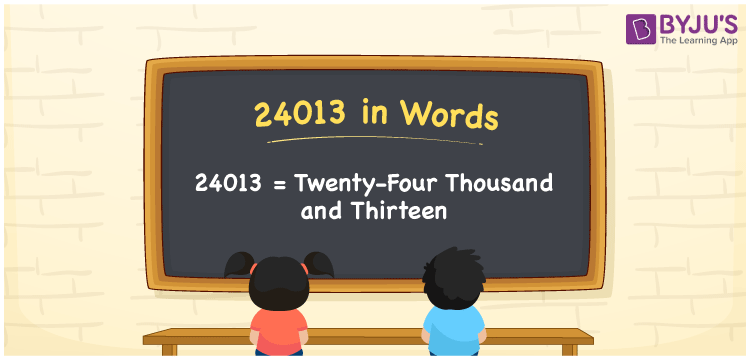# 24013 in Words

24013 in words is written as Twenty-four thousand thirteen. In both the International System of Numerals and the Indian System of Numerals, 24013 is written as Twenty-four thousand thirteen. The number 24013 is a Cardinal Number as it denotes some quantity. For example, “the mobile phone costs 24013 rupees”.

 24013 in Words Twenty-four thousand thirteen Twenty-four thousand thirteen in Number 24013

## 24013 in English Words

24013 in English words is read as “Twenty-four thousand thirteen”.## How to Write 24013 in Words?

To write 24013 in words, we shall use the place value chart. In the place value chart, put 2 in the ten thousands, 4 in the thousands, 0 in the hundreds, 1 in the tens and 3 in the ones. Let us make a place value chart to write the number 24013 in words.

 Ten Thousands Thousands Hundreds Tens Ones 2 4 0 1 3

Thus, we can write the expanded form as

2 × Ten Thousand + 4 × Thousand + 0 × Hundred + 1 × Ten + 3 × One

= 2 × 10000 + 4 × 1000 + 0 × 100 + 1 × 10 + 3 × 1

= 20000 + 4000 + 0 +10 + 3

= 24013

= Twenty-four thousand thirteen.

24013 is a natural number, the successor of 24012 and the predecessor of 24014.

24013 in words – Twenty-four thousand thirteen

• Is 24013 an odd number? – Yes
• Is 24013 an even number? – No
• Is 24013 a perfect square number? – No
• Is 24013 a perfect cube number? – No
• Is 24013 a prime number? – No
• Is 24013 a composite number? – Yes

## Frequently Asked Questions on 24013 in Words

Q1

### How to write 24013 in words?

24013 in words is written as Twenty-four thousand thirteen.
Q2

### How to write 24013 in the International and Indian System of Numerals?

In both, the system of numerals, 24013 in words, is written as Twenty-four thousand thirteen.
Q3

### How to write 24013 in a place value chart?

In the place value chart, write 2 in the ten thousands, 4 in the thousands, 0 in the hundreds, 1 in the tens and 3 in the ones, respectively.